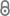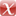# A Loosely Self-stabilizing Protocol for Randomized Congestion Control with Logarithmic Memory

M. Feldmann, T. Götte, C. Scheideler, in: Proceedings of the 21st International Symposium on Stabilization, Safety, and Security of Distributed Systems (SSS), 2019.main.pdf 428.91 KB
Conference Paper | English
Author
Department
Abstract
We consider congestion control in peer-to-peer distributed systems. The problem can be reduced to the following scenario: Consider a set $V$ of $n$ peers (called \emph{clients} in this paper) that want to send messages to a fixed common peer (called \emph{server} in this paper). We assume that each client $v \in V$ sends a message with probability $p(v) \in [0,1)$ and the server has a capacity of $\sigma \in \mathbb{N}$, i.e., it can recieve at most $\sigma$ messages per round and excess messages are dropped. The server can modify these probabilities when clients send messages. Ideally, we wish to converge to a state with $\sum p(v) = \sigma$ and $p(v) = p(w)$ for all $v,w \in V$. We propose a \emph{loosely} self-stabilizing protocol with a slightly relaxed legitimate state. Our protocol lets the system converge from \emph{any} initial state to a state where $\sum p(v) \in \left[\sigma \pm \epsilon\right]$ and $|p(v)-p(w)| \in O(\frac{1}{n})$. This property is then maintained for $\Omega(n^{\mathfrak{c}})$ rounds in expectation. In particular, the initial client probabilities and server variables are not necessarily well-defined, i.e., they may have arbitrary values. Our protocol uses only $O(W + \log n)$ bits of memory where $W$ is length of node identifiers, making it very lightweight. Finally we state a lower bound on the convergence time an see that our protocol performs asymptotically optimal (up to some polylogarithmic factor).
Publishing Year
Proceedings Title
Proceedings of the 21st International Symposium on Stabilization, Safety, and Security of Distributed Systems (SSS)
LibreCat-ID

### Cite this

Feldmann M, Götte T, Scheideler C. A Loosely Self-stabilizing Protocol for Randomized Congestion Control with Logarithmic Memory. In: Proceedings of the 21st International Symposium on Stabilization, Safety, and Security of Distributed Systems (SSS). Lecture Notes in Computer Science. ; 2019.
Feldmann, M., Götte, T., & Scheideler, C. (2019). A Loosely Self-stabilizing Protocol for Randomized Congestion Control with Logarithmic Memory. In Proceedings of the 21st International Symposium on Stabilization, Safety, and Security of Distributed Systems (SSS).
@inproceedings{Feldmann_Götte_Scheideler_2019, series={Lecture Notes in Computer Science}, title={A Loosely Self-stabilizing Protocol for Randomized Congestion Control with Logarithmic Memory}, booktitle={Proceedings of the 21st International Symposium on Stabilization, Safety, and Security of Distributed Systems (SSS)}, author={Feldmann, Michael and Götte, Thorsten and Scheideler, Christian}, year={2019}, collection={Lecture Notes in Computer Science} }
Feldmann, Michael, Thorsten Götte, and Christian Scheideler. “A Loosely Self-Stabilizing Protocol for Randomized Congestion Control with Logarithmic Memory.” In Proceedings of the 21st International Symposium on Stabilization, Safety, and Security of Distributed Systems (SSS). Lecture Notes in Computer Science, 2019.
M. Feldmann, T. Götte, and C. Scheideler, “A Loosely Self-stabilizing Protocol for Randomized Congestion Control with Logarithmic Memory,” in Proceedings of the 21st International Symposium on Stabilization, Safety, and Security of Distributed Systems (SSS), 2019.
Feldmann, Michael, et al. “A Loosely Self-Stabilizing Protocol for Randomized Congestion Control with Logarithmic Memory.” Proceedings of the 21st International Symposium on Stabilization, Safety, and Security of Distributed Systems (SSS), 2019.
Main File(s)
File Name
main.pdf 428.91 KB
Access LevelClosed Access
2019-09-11T11:20:16Z

### Export

Marked Publications

Open Data LibreCat

### SourcesarXiv 1909.04544